Related Articles
Introduction to Explainable AI(XAI) using LIME
• Difficulty Level : Medium
• Last Updated : 04 Mar, 2021

Motivating Explainable AI

The vast field of Artificial Intelligence(AI) has experienced enormous growth in recent years. With newer and more complex models coming each year, AI models have started to surpass human intellect at a pace that no one could have predicted. But as we get more accurate and precise results, it’s becoming harder to explain the reasoning behind the complex mathematical decisions these models take. This mathematical abstraction also doesn’t help the users maintain their trust in a particular model’s decisions.

e.g., Say a Deep Learning model takes in an image and predicts with 70% accuracy that a patient has lung cancer. Though the model might have given the correct diagnosis, a doctor can’t really advise a patient confidently as he/she doesn’t know the reasoning behind the said model’s diagnosis.

Here’s where Explainable AI(or more popularly known as XAI) comes in! Explainable AI collectively refers to techniques or methods, which help explain a given AI  model’s decision-making process. This newly found branch of AI has shown enormous potential, with newer and more sophisticated techniques coming each year. Some of the most famous XAI techniques include SHAP (Shapley Additive exPlanations), DeepSHAP, DeepLIFT, CXplain, and LIME. This article covers LIME in detail.

Introducing LIME(or Local  Interpretable Model-agnostic Explanations)

The beauty of LIME its accessibility and simplicity. The core idea behind LIME though exhaustive is really intuitive and simple! Let’s dive in and see what the name itself represents:

• Model agnosticism refers to the property of LIME using which it can give explanations for any given supervised learning model by treating as a ‘black-box’ separately. This means that LIME can handle almost any model that exists out there in the wild!
• Local explanations mean that LIME gives explanations that are locally faithful within the surroundings or vicinity of the observation/sample being explained.

Though LIME limits itself to supervised Machine Learning and Deep Learning models in its current state, it is one of the most popular and used XAI methods out there. With a rich open-source API, available in R and Python, LIME boasts a huge user base, with almost 8k stars and 2k forks on its Github repository.

How LIME works?

Broadly speaking, when given a prediction model and a test sample, LIME does the following steps:

• Sampling and obtaining a surrogate dataset: LIME provides locally faithful explanations around the vicinity of the instance being explained. By default, it produces 5000 samples(see the num_samples variable) of the feature vector following the normal distribution. Then it obtains the target variable for these 5000 samples using the prediction model, whose decisions it’s trying to explain.
• Feature Selection from the surrogate dataset: After obtaining the surrogate dataset, it weighs each row according to how close they are from the original sample/observation. Then it uses a feature selection technique like Lasso to obtain the top important features.

LIME also employs a Ridge Regression model on the samples using only the obtained features. The outputted prediction should theoretically be similar in magnitude to the one outputted by the original prediction model. This is done to stress the relevance and importance of these obtained features.

We won’t really dive into the technical and mathematical details behind the internals of LIME in this article. Still, you can go through the base research paper if you’re interested in it. Now, onto the more interesting part, the code!

Installing LIME

Coming to the installation part, we can use either pip or conda to install LIME in Python.

`pip install lime`

or

`conda install -c conda-forge lime`

Before going ahead, here are some key pointers that would help gain a much better understanding of the whole workflow surrounding LIME.

### Dataset Description:

LIME in its current state is only able to give explanations for the following type of datasets:

1. Tabular datasets (lime.lime_tabular.LimeTabularExplainer): eg: Regression, classification datasets
2. Image related datasets (lime.lime_image.LimeImageExplainer)
3. Text related datasets (lime.lime_text.LimeTextExplainer)

Since this is an introductory article, we’ll keep things simple and go ahead with a tabular dataset. More specifically, we’ll be using the Boston House Pricing dataset for our analysis. We’ll be using the Scikit-Learn utility for loading the dataset.

### Prediction Model Used:

As LIME is model agnostic in nature, it can handle almost any model thrown at it. To stress this fact, we’ll be using an Extra-trees regressor through the Scitkit-learn utility as our prediction model whose decisions we’re trying to investigate.

### Brief Introduction to LimeTabularExplainer

As explained above, we’ll be using a tabular dataset for our analysis. To tackle such datasets, LIME’s API offers the LimeTabularExplainer.

Syntax: lime.lime_tabular.LimeTabularExplainer(training_data, mode,  feature_names, verbose)

Parameters:

• training_data – 2d array consisting of the training dataset
• mode – Depends on the problem; “classification” or “regression”
• feature_names – list of titles corresponding to the columns in the training dataset. If not mentioned, it uses the cloumn indices.
• verbose – if true, print local prediction values from the regression model trained on the samples using only the obtained features

Once instantiated, we’ll use a method from the defined explainer object to explain a given test sample.

Syntax: explain_instance(data_row, predict_fn, num_features=10, num_samples=5000)

Parameters:

• data_row – 1d array containing values corresponding to the test sample being explained
• predict_fn – Prediction function used by the prediction model
• num_features – maximum number of features present in explanation
• num_samples – size of the neighborhood to learn the linear model

For the sake of brevity and conciseness, only some of the arguments have been mentioned in the above two syntaxes. The rest of the arguments, most of which default to some cleverly optimized values, can be checked out by the interested reader at the official LIME documentation.

Workflow

1. Data preprocessing
2. Training an Extra-trees regressor on the dataset
3. Obtaining explanations for a given test sample

Analysis

## Python

 `# Importing the necessary libraries``import` `numpy as np``import` `matplotlib.pyplot as plt``import` `pandas as pd` `# Loading the dataset using sklearn``from` `sklearn.datasets ``import` `load_boston``data ``=` `load_boston()` `# Displaying relevant information about the data``print``(data[``'DESCR'``][``200``:``1420``])`

Output: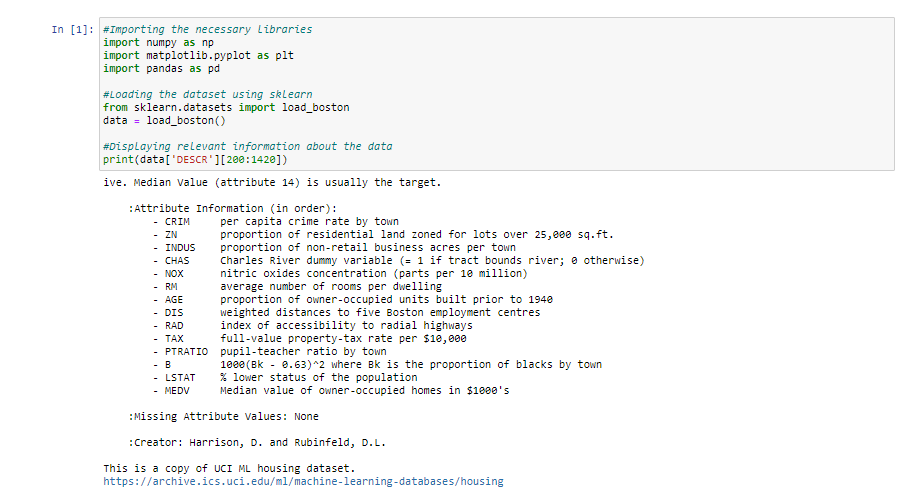Jupyter notebook output of above code

## Python

 `# Separating data into feature variable X and target variable y respectively``from` `sklearn.model_selection ``import` `train_test_split``X ``=` `data[``'data'``]``y ``=` `data[``'target'``]` `# Extracting the names of the features from data``features ``=` `data[``'feature_names'``]` `# Splitting X & y into training and testing set``X_train, X_test, y_train, y_test ``=` `train_test_split(``    ``X, y, train_size``=``0.90``, random_state``=``50``)` `# Creating a dataframe of the data, for a visual check``df ``=` `pd.concat([pd.DataFrame(X), pd.DataFrame(y)], axis``=``1``)``df.columns ``=` `np.concatenate((features, np.array([``'label'``])))``print``(``"Shape of data ="``, df.shape)` `# Printing the top 5 rows of the dataframe``df.head()`

Output: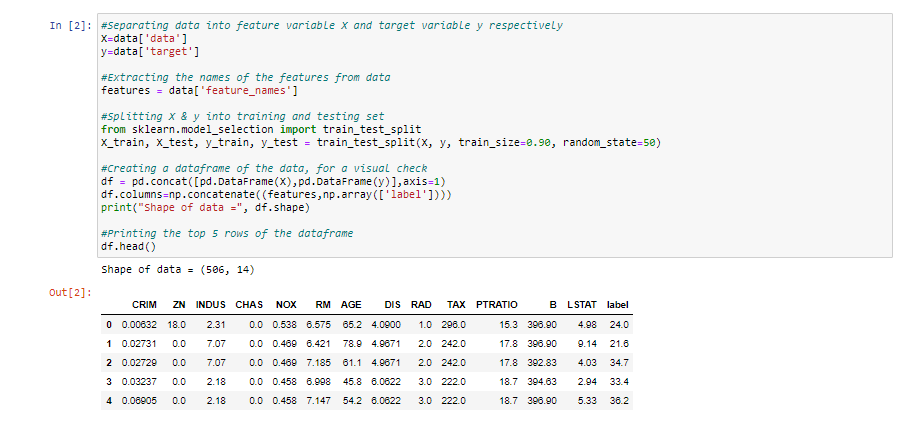Jupyter notebook output of above code

## Python

 `# Instantiating the prediction model - an extra-trees regressor``from` `sklearn.ensemble ``import` `ExtraTreesRegressor``reg ``=` `ExtraTreesRegressor(random_state``=``50``)` `# Fitting the predictino model onto the training set``reg.fit(X_train, y_train)` `# Checking the model's performance on the test set``print``(``'R2 score for the model on test set ='``, reg.score(X_test, y_test))`

Output: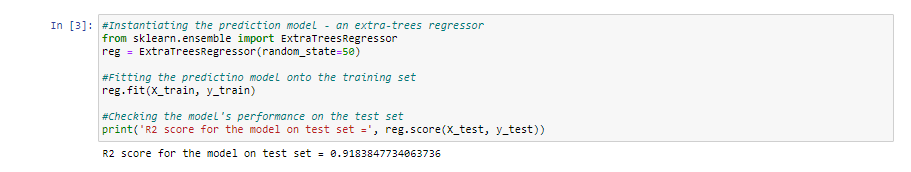Jupyter notebook output of above code

## Python

 `# Importing the module for LimeTabularExplainer``import` `lime.lime_tabular` `# Instantiating the explainer object by passing in the training set, and the extracted features``explainer_lime ``=` `lime.lime_tabular.LimeTabularExplainer(X_train,``                                                        ``feature_names``=``features,``                                                        ``verbose``=``True``, mode``=``'regression'``)`

### 5. Getting explanations by calling the explain_instance() method

• Suppose we want to explore the prediction model’s reasoning behind the prediction it gave for the i’th test vector.
• Moreover, say we want to visualize the top k features which led to this reasoning.

#### 5.1 Explaining the decisions for i=10, k=5

We’re basically asking LIME to explain the decisions behind the predictions for the 10th test vector by displaying the top 5 features which contributed towards the said model’s prediction.

## Python

 `# Index corresponding to the test vector``i ``=` `10` `# Number denoting the top features``k ``=` `5` `# Calling the explain_instance method by passing in the:``#    1) ith test vector``#    2) prediction function used by our prediction model('reg' in this case)``#    3) the top features which we want to see, denoted by k``exp_lime ``=` `explainer_lime.explain_instance(``    ``X_test[i], reg.predict, num_features``=``k)` `# Finally visualizing the explanations``exp_lime.show_in_notebook()`

Output: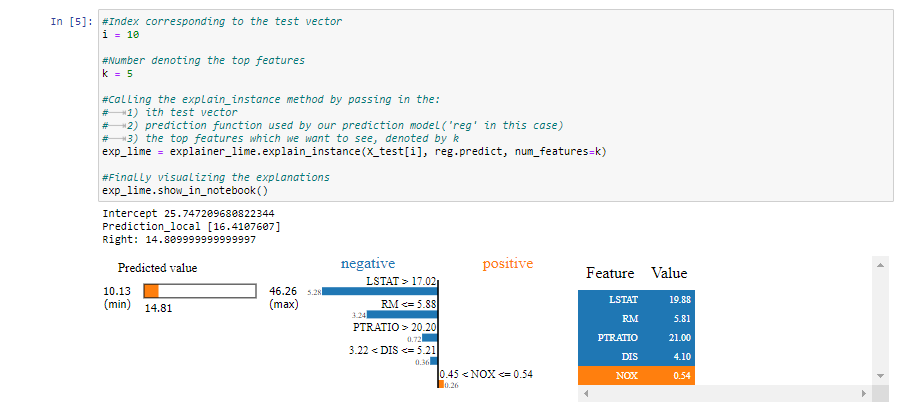Jupyter notebook output of above code

Interpreting the output

There’s plenty of information that LIME outputs! Let’s go step by step and interpret what it’s trying to convey

• First off, we see three values just above the visualizations:
1. Right: This denotes the prediction given by our prediction model(an extra-trees regressor in this case) for the given test vector.
2. Prediction_local: This denotes the value outputted by a linear model trained on the perturbed samples(obtained by sampling around the test vector following a normal distribution) and using only the top k features outputted by LIME.
3. Intercept: The intercept is the constant part of the prediction given by the above linear model’s prediction for the given test vector.• Coming to the visualizations, we can see the colors blue and orange, depicting negative and positive associations, respectively.
• To interpret the above results, we can conclude that the relatively lower price value(depicted by a bar on the left) of the house depicted by the given vector can be attributed to the following socio-economic reasons:
• the high value of LSTAT indicating the lower status of a society in terms of education and unemployability
• the high value of PTRATIO indicating the high value of the number of students per teacher
• the high value of DIS indicating the high value of the distance from employment centers.
• the low value of RM indicating the less amount of room per dwelling
• We can also see that the low value of NOX indicates that the low amount of nitric oxide concentration in the air has increased the house’s value to a small extent.

We can see how easy it has become to correlate the decisions taken by a relatively complex prediction model(an extra-trees regressor) in an interpreatable and meaningful way. Let’s try this exercise on one more test vector!

#### 5.2 Explaining the decisions for i=47, k=5

Here again we’re asking LIME to explain the decisions behind the predictions for the 47th test vector by displaying the top 5 features which contributed towards the said model’s prediction

## Python

 `# Index corresponding to the test vector``i ``=` `47` `# Number denoting the top features``k ``=` `5` `# Calling the explain_instance method by passing in the:``#    1) ith test vector``#    2) prediction function used by our prediction model('reg' in this case)``#    3) the top features which we want to see, denoted by k``exp_lime ``=` `explainer_lime.explain_instance(``    ``X_test[i], reg.predict, num_features``=``k)` `# Finally visualizing the explanations``exp_lime.show_in_notebook()`

Output: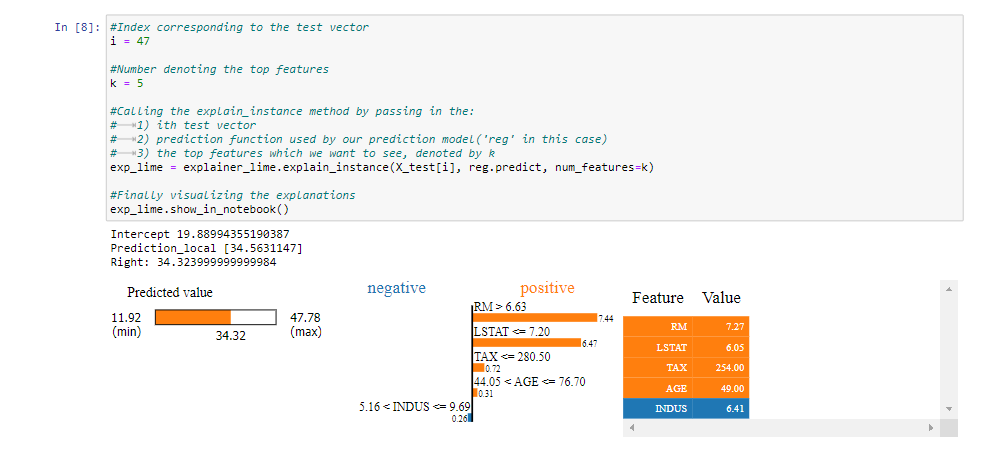Jupyter notebook output of above code

Interpreting the output:

• From the visualizations, we can conclude that the relatively higher price value(depicted by a bar on the left) of the house depicted by the given vector can be attributed to the following socio-economic reasons:
• The low value of LSTAT indicating the grand status of a society in terms of education and employability
• The high value of RM indicating the high numbers of room per dwelling
• The low value of TAX indicating the low tax-rate of the property
• The low value of AGE which depicts the newness of the establishment
• We can also see that the average value of INDUS, which indicates that the low number of non-retails near the society, has decreased the value of the house to a small extent.

Summary:

This article is a brief introduction to Explainable AI(XAI) using LIME in Python. It’s evident how beneficial LIME could give us a much profound intuition behind a given black-box model’s decision-making process while providing solid insights on the inherent dataset. This makes LIME a useful resource for both AI researchers and data scientists alike!

References:

1. https://lime-ml.readthedocs.io/en/latest/
2. https://scikit-learn.org/stable/modules/generated/sklearn.ensemble.ExtraTreesRegressor.html
3. https://scikit-learn.org/0.16/modules/generated/sklearn.datasets.load_boston.html#sklearn.datasets.load_boston
4. Marco Tulio Ribeiro, Sameer Singh, and Carlos Guestrin. ”why should I trust you?”: Explaining the predictions of any classifier. In Proceedings of the 22nd ACM SIGKDD International Conference on Knowledge Discovery and Data Mining, page 1135–1144. Association for Computing Machinery, 2016.My Personal Notes arrow_drop_up